• 5
•
•
•
•
•
•
•
5
Shares

# WORK AND TIME PROBLEMS TRICKS IN HINDI1). If a man can do a piece of work in D days, then his work in one day =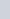2). If a man’s work in 1 day is 1/D, then the time taken to complete the work is = D days

3). If one person is n times faster than another person, then –

*.The ratio of work done by first and second person = n:1

*. Time is taken by a first and second person to finish the work = 1:n

4). If A can do a work in x days and B in y days, then together they will do that work in =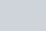5). If A and B can do a piece of work in x days and A alone can do that work in Y days, then B alone will do that work in=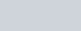6). If A, B, and C can do a piece of work in x, y, and z days respectively, then all three together will complete that work in =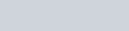7). If A and B can do a piece of work in x and y days, and working together earn Rs x wages, then –
*. part of A =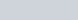*.B’s part =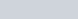8). If A and B can do a work in x days, B and C can do the same work in y days and C and A can do the same work in z days, then all three together will do that work =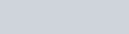9). If A and B can complete a work in x and y days respectively, they start working together, and A leaves after t days, then the time taken to complete the work is =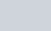10). If A and B can complete a work in x and y days respectively, they start working together but A left the work t days before the completion of the work, then the time taken to complete the work is =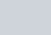11). A, B, and C can do work in x,y, and z days respectively, all three started working together, and A and B left the work t1 day before and t2 days before the end of the work, then the time is taken to complete the work =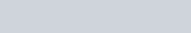12). If a man and b-boys can do a piece of work in x days, then c men and d boys will do that work in=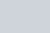13). If a man can do a piece of work in x days and b-boys can do the same work in y days, then the time taken by c men and d boys to do that work =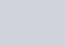14). If the ratio of men to do work is m: n, then the ratio of their time = n: m

15). If a place has food for x persons for a day, and after b-days, y persons come/leave, then the rest of the food supply for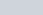persons will be for days=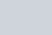16). Some persons complete work in x days, but after sometimes a person does not participate in completing the work due to some reason, then the work is completed in y days, the number of persons at the beginning of the work =17). If A can do a piece of work in x days, and B can do the work y% faster than A, then the time taken by B to do that work =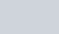18). Some persons can complete a work in x days, if m persons leave the work or come in between, then it takes y days more/less to complete the work, then the number of persons, in the beginning, is =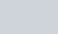19). If x1 persons working t1 hours per day do w1 work in n1 days, and x2 persons working t2 hours per day doing w2 work in n2 days, then the relation of the following =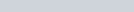20). If A can do a piece of work in x days, B can do the same work in y days, and C can do the same work in z days, then their works in one day.=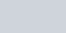21). A person can do a work in D days, if a persons were less then, d days more would have taken to complete the work, then the number of persons =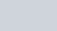22). If a1 persons and b1 boys together can complete a work in x days, and a2 persons and b2 boys together complete the same work in y days, then the relation of the following-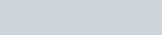23). If the work done by a1 men and b1 boys together per day is n times the work done by a2 men and b2 boys together per day, then-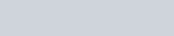24). If X and Y take a and b-days more to do a piece of work than the time taken by X and Y together, respectively, then the time taken by X and Y to work together is =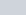25). X and Y can complete a work in a and b-days separately, both start working together, only then X or Y leaves the work x days before the completion of the work, then the time is taken to finish the work-
*. for x*. for y26). X and Y can complete a work separately in a and b-days, both start working together, but after some time X leaves the work, and Y finishes the remaining work in x days, Then the time after which X left the work =